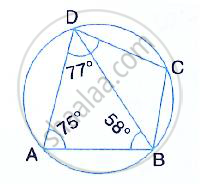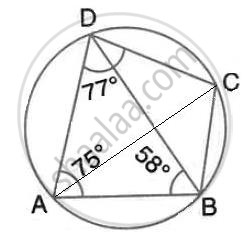Share

In the Figure, Given Below, Abcd is a Cyclic Quadrilateral in Which ∠Bad = 75°; ∠Abd = 58° and ∠Adc = 77°. Find: (I) ∠Bdc, (Ii) ∠Bcd, (Iii) ∠Bca. - ICSE Class 10 - Mathematics

Question

In the figure, given below, ABCD is a cyclic quadrilateral in which ∠BAD = 75°; ∠ABD = 58°
and ∠ADC = 77°. Find:

(i) ∠BDC, (ii) ∠BCD, (iii) ∠BCA.Solution(i) By angle – sum property of triangle ABD,
∠ADB= 180° -75° -58° = 47°
∴ ∠BDC =∠ADC - ∠ADB = 77° - 47° = 30°

(ii) ∠BAD + ∠BCD = 180°
(Sum of opposite angles of a cyclic quadrilateral is 180° )
⇒ ∠BCD= 180° - 75° - 105°

(iii) ∠BDA = ∠ADB = 47°
(Angle subtended by the same chord on the circle are equal)

Is there an error in this question or solution?
Solution In the Figure, Given Below, Abcd is a Cyclic Quadrilateral in Which ∠Bad = 75°; ∠Abd = 58° and ∠Adc = 77°. Find: (I) ∠Bdc, (Ii) ∠Bcd, (Iii) ∠Bca. Concept: Cyclic Properties.
S